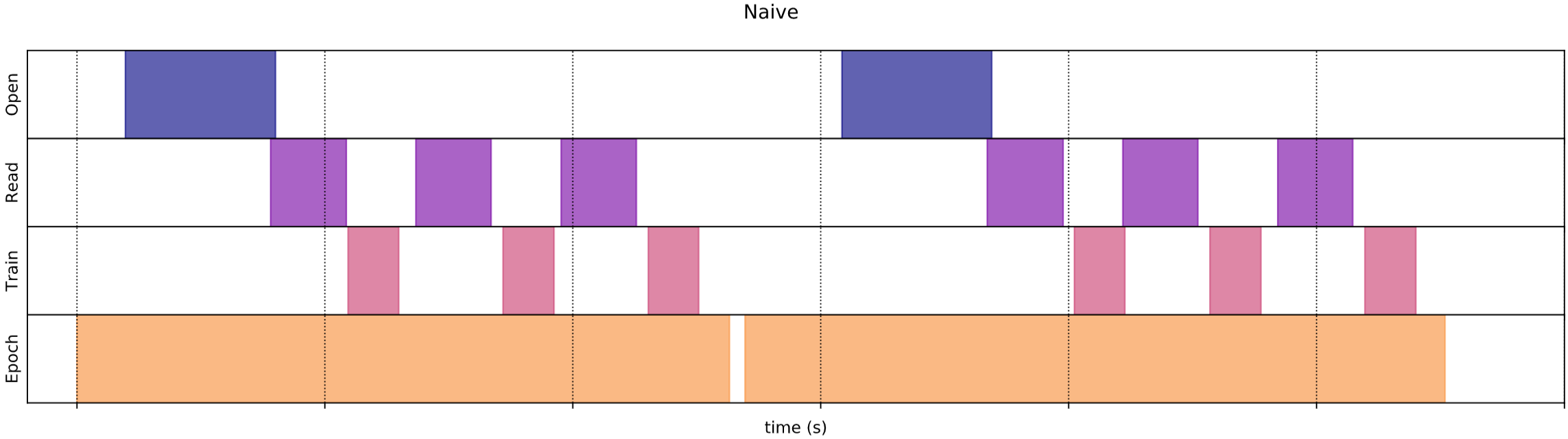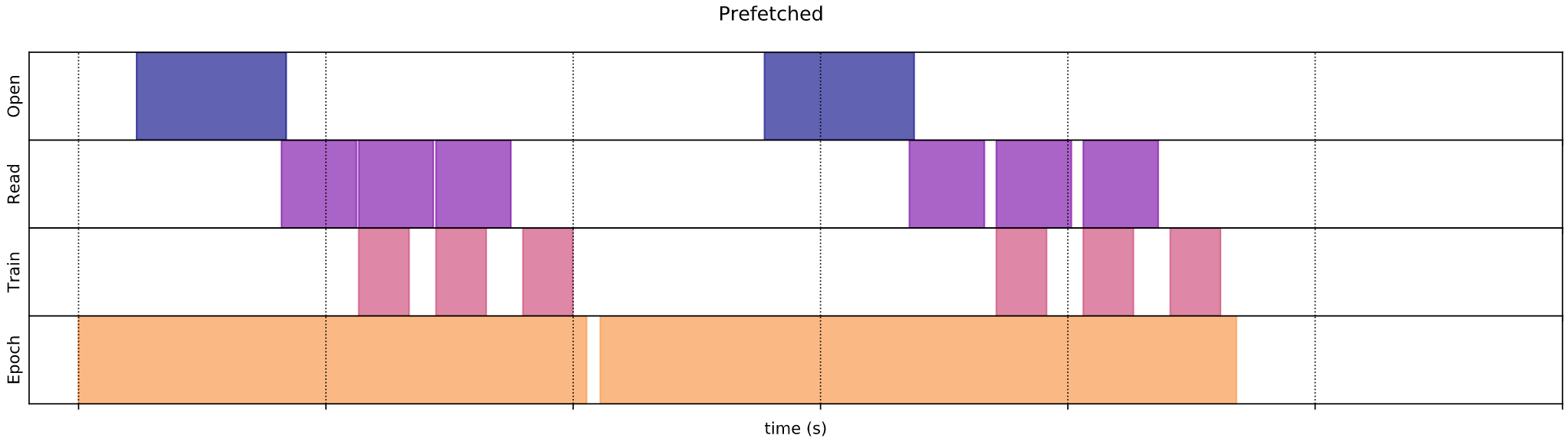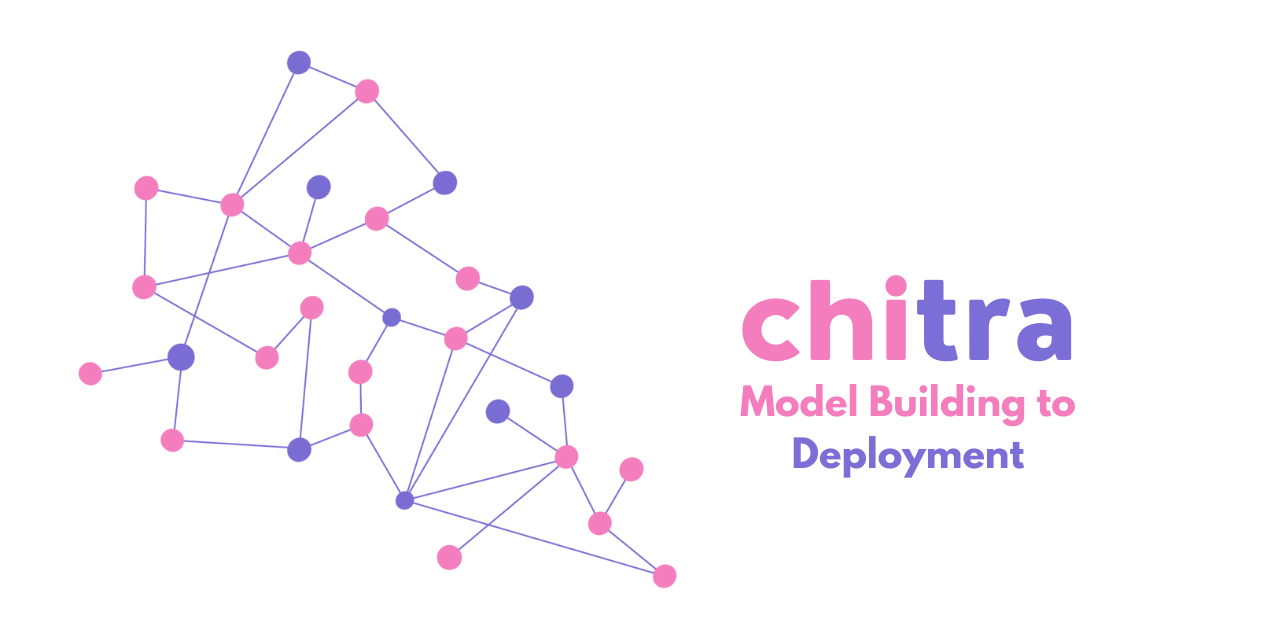# tf.data: Creating data input pipelines

Building scalabale data input pipeline with Tensorflow tf.data.
Tensorflow
Published

April 8, 2020

Are you not able to load your NumPy data into memory? Does your model have to wait for data to be loaded after each epoch? Is your Keras DataGenerator slow?

TensorFlow tf.data API allows building complex input pipelines. It easily handles a large amount of data and can read different formats of data while allowing complex data transformations.

## Why do we need tf.data?

A training step involves the following steps: 1. File reading 2. Fetch or parse data 3. Data transformation 4. Using the data to train the model.source: Tensorflow

If you have a large amount of data and you’re unable to load it into the memory, you may want to use Generators. But Generators has limited portability and scalability.

After every epoch, you will wait for data to be transformed into a consumable format by the model and during that time your model sits idle, not doing any training. This leads to low CPU and GPU utilization.

One solution to handle this is to prefetch your data in advance and you won’t have to wait for data to be loaded.source: Tensorflow

tf.data is a data input pipeline building API than you can use to easily build your data pipeline. Whether you want to read data from local files or even if your data is stored remotely.

To train an image classification model, we create a CNN model and feed our data to the model. I want to train a Cats vs Dogs classifier and my data is stored in the following folder structure.

``````data
└── train
├── cat -> contains images of cats
└── dog -> contains images of dogs*``````

We first find the path of all the images-

``````from glob import glob
import tensorflow as tf

image_path_list = glob('data/train/*/*.jpg')
data = tf.data.Dataset.list_files(image_path_list)``````

`tf.data.Dataset.list_files` converts the list returned by glob method to the Dataset object. Now, we will load the images and their class.

``````def load_images(path):

image = tf.io.decode_image(image)
label = tf.strings.split(path, os.path.sep)[-2]

return image, label

So, the data object now has images and labels. But this is not it, we will have to resize the image, preprocess and apply transformations.

``````def preprocess(image, label):
image = tf.image.resize(image, (IMG_HEIGHT, IMG_WIDTH))
image = tf.image.random_flip_left_right(image)
image = tf.image.random_flip_up_down(image)

image /= 255.
image -= 0.5

return image, label

data = data.map(preprocess)``````

I have created a small library named Chitra, based on tf.data that can be used to skip all these steps.

``````from chitra import dataloader as dl
path = './data/train'

train_dl = dl.Clf()
data = train_dl.from_folder(path, target_shape=(224, 244), shuffle = True)

# to visualize the data
train_dl.show_batch(6, figsize=(6,6))``````

You can just specify the path of your data and it will be loaded with the target size.> You can find my code at https://github.com/aniketmaurya/chitra